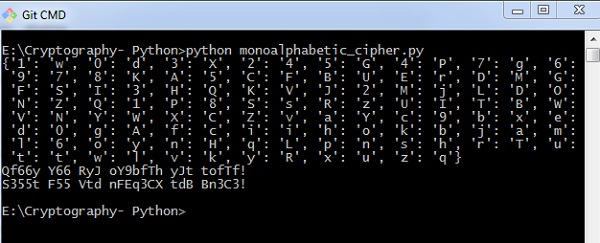# Hacking Monoalphabetic Cipher

In this chapter, you will learn about monoalphabetic cipher and its hacking using Python.

## Monoalphabetic Cipher

A Monoalphabetic cipher uses a fixed substitution for encrypting the entire message. A monoalphabetic cipher using a Python dictionary with JSON objects is shown here −

```monoalpha_cipher = {
'a': 'm',
'b': 'n',
'c': 'b',
'd': 'v',
'e': 'c',
'f': 'x',
'g': 'z',
'h': 'a',
'i': 's',
'j': 'd',
'k': 'f',
'l': 'g',
'm': 'h',
'n': 'j',
'o': 'k',
'p': 'l',
'q': 'p',
'r': 'o',
's': 'i',
't': 'u',
'u': 'y',
'v': 't',
'w': 'r',
'x': 'e',
'y': 'w',
'z': 'q',
' ': ' ',
}```

With help of this dictionary, we can encrypt the letters with the associated letters as values in JSON object. The following program creates a monoalphabetic program as a class representation which includes all the functions of encryption and decryption.

```from string import letters, digits
from random import shuffle

def random_monoalpha_cipher(pool = None):
if pool is None:
pool = letters + digits
original_pool = list(pool)
shuffled_pool = list(pool)
shuffle(shuffled_pool)
return dict(zip(original_pool, shuffled_pool))

def inverse_monoalpha_cipher(monoalpha_cipher):
inverse_monoalpha = {}
for key, value in monoalpha_cipher.iteritems():
inverse_monoalpha[value] = key
return inverse_monoalpha

def encrypt_with_monoalpha(message, monoalpha_cipher):
encrypted_message = []
for letter in message:
encrypted_message.append(monoalpha_cipher.get(letter, letter))
return ''.join(encrypted_message)

def decrypt_with_monoalpha(encrypted_message, monoalpha_cipher):
return encrypt_with_monoalpha(
encrypted_message,
inverse_monoalpha_cipher(monoalpha_cipher)
)```

This file is called later to implement the encryption and decryption process of Monoalphabetic cipher which is mentioned as below −

```import monoalphabeticCipher as mc

cipher = mc.random_monoalpha_cipher()
print(cipher)
encrypted = mc.encrypt_with_monoalpha('Hello all you hackers out there!', cipher)
decrypted = mc.decrypt_with_monoalpha('sXGGt SGG Nt0 HSrLXFC t0U UHXFX!', cipher)

print(encrypted)
print(decrypted)```

### Output

You can observe the following output when you implement the code given above −Thus, you can hack a monoalphabetic cipher with specified key value pair which cracks the cipher text to actual plain text.